Dr. Barry Haworth
University of Louisville
Department of Economics
Economics 401

### A Utility Max Applicationof the Implicit Function Theorem

Assume that a consumer named Homer Simpson consumes varying amounts of Duff beer and pork rinds

Let:

• units of beer consumed = B
• units of pork rinds consumed = R

Homer derives his utility from consuming these goods in accordance with the following utility function (where U = utility):

 (1) U = f(B,R)

Homer's purchasing decision is limited by the following budget constraint (where pi is the price of good i, and I is Homer's income):

 (2) pBB + pRR = I

Note that (2) can be rearranged to become:

 (2a)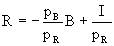Utility maximization leads us to the following equilibrium condition (which says that the slope of the indifference curve equals the slope of the budget constraint):

 (3)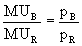(where MUi = marginal utility of good i; which equals the derivative of the utility function with respect to good i)

Let us first take the total derivative of (1), the utility function. Upon doing so, we have:

 (4)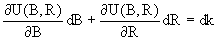(where k is a constant equal to some overall level of utility, such that k ³ 0)

Dividing both sides of (4) by dB yields:

 (5)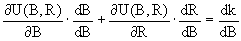Because dB/dB = 1, and dk/dB = 0, we can simplify (5) to get:

 (5a)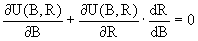Solving (5a) for dR/dB yields:

 (5a)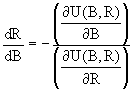At this point, we need to stop and ask what we've got thus far. In doing so, let's recall a couple of points made above. First, we note that the marginal utility of good i can be expressed as the first derivative of the utility function taken with respect to good i. Second, we note that an indifference curve's slope is equal (in the two-good case) to the ratio of the marginal utilities.

Because the righthand side of (6) involves the ratio of two derivatives of the utility function (each taken with respect to one of the goods consumed by Homer), the righthand side of (6) must be the slope of Homer's indifference curve. If the slope of Homer's indifference curve was set equal to the slope of his budget constraint, then we would have the consumer equilibrium expression given in (3).

To take the actual derivatives just mentioned, however, we need to assume a functional form for the utility function in (1). Let's assume a linear (additive) utility function for this example, the function given below (where q is a parameter that's greater than zero, a is a parameter that's between 0 and 1, and ln(i) = natural log of good i):

 (1a) U = q + aln(B) + (1 - a)ln(R)

If we take the derivatives described in (6) and substitute those derivatives into (3), then we have (recall that if y = ln(x), then dy/dx = 1/x):

 (7)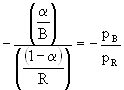The two equations which describe the tangency point between Homer's indifference curve and his budget constraint are (7) and (2a). Using these equations together, we can solve for B* and R*. In their present form, those solutions are: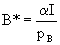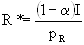If we wish to go further and assume numerical values for the parameters in this model, then we could assume the following:

 a = 0.5 pB = \$4 q = 100 pR = \$2 I = \$200

Substituting into our solution above, the numerical values for B* and R* are:

B* = 25
R* = 50

These are the amounts of beer and pork rinds that will give Homer his maximum utility.